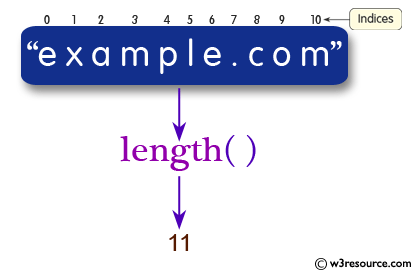# Java String: length() Method

## public int length()

The length() method is used to get the length of this string. The length is equal to the number of Unicode code units in the string.

Java Platform: Java SE 8

Syntax:

`length()`

Return Value: the length of the sequence of characters represented by this object.

Return Value Type: int

Pictorial presentation of Java String length() MethodExample: Java String length() Method

The following example shows the usage of java String() method.

``````public class Example {
public static void main(String[] args)
{
String str = "example.com";
// Get the length of str.
int len = str.length();
System.out.println();
System.out.println("The string length of '"+str+"' is: "+len);
System.out.println();
}
}
```
```

Output:

```The string length of 'example.com' is: 11
```

Java Code Editor :

Previous:lastIndexOf Method
Next:matches Method

﻿